# Electric Field Contents Basic Concept Calculating electric Field

• Slides: 27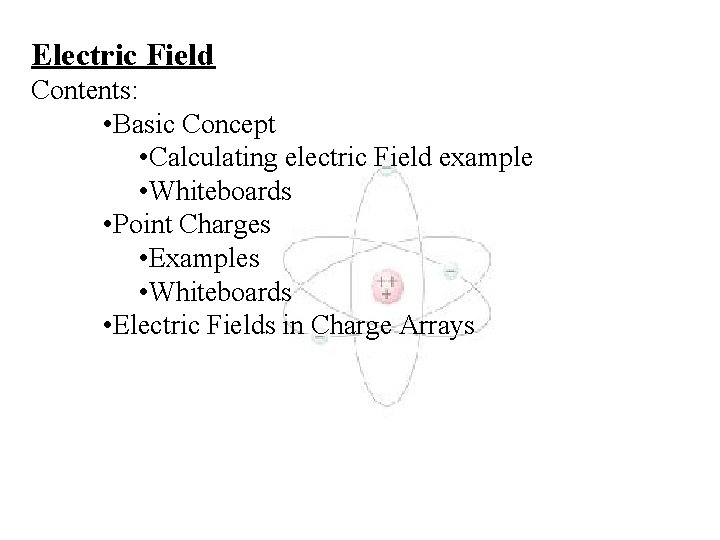Electric Field Contents: • Basic Concept • Calculating electric Field example • Whiteboards • Point Charges • Examples • Whiteboards • Electric Fields in Charge Arrays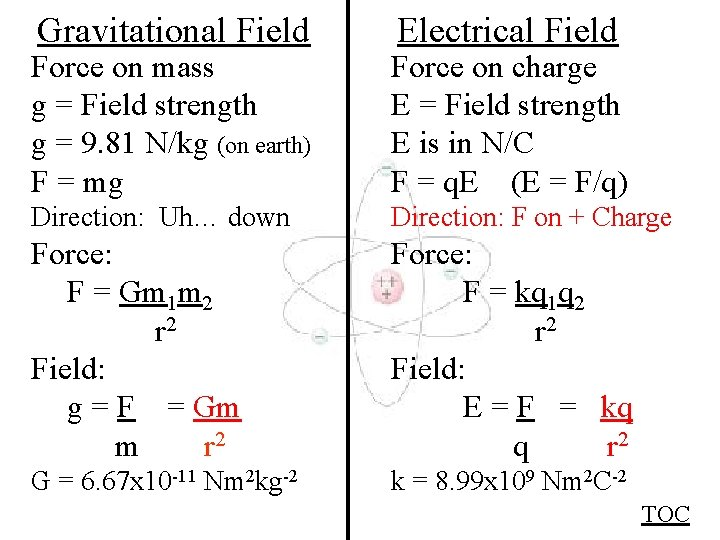Gravitational Field Electrical Field Force on mass g = Field strength g = 9. 81 N/kg (on earth) F = mg Force on charge E = Field strength E is in N/C F = q. E (E = F/q) Direction: Uh… down Direction: F on + Charge Force: F = Gm 1 m 2 r 2 Field: g = F = Gm m r 2 Force: F = kq 1 q 2 r 2 Field: E = F = kq q r 2 G = 6. 67 x 10 -11 Nm 2 kg-2 k = 8. 99 x 109 Nm 2 C-2 TOCElectric Field Example 1 - A +125 C charge experiences a force to the right of. 0175 N. What is the Electric field, and its direction? E = F/q =. 0175 N/125 x 10 -6 C = 140 N/C to the right Direction: +q Force This Way E -q TOC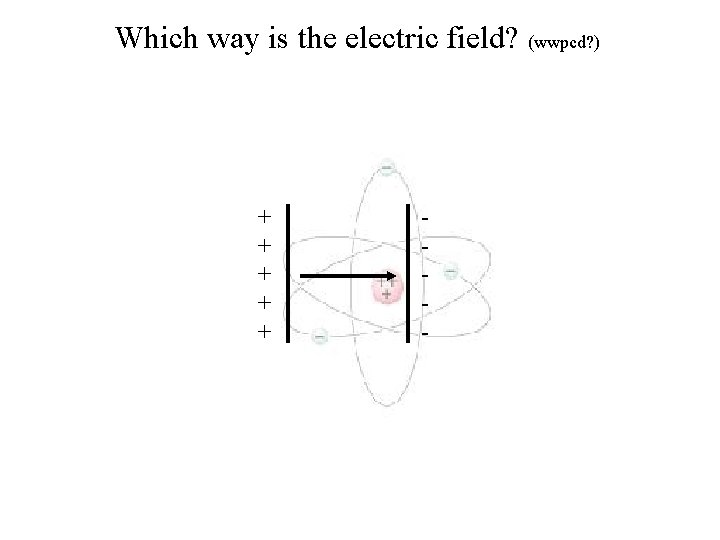Which way is the electric field? (wwpcd? ) + + + -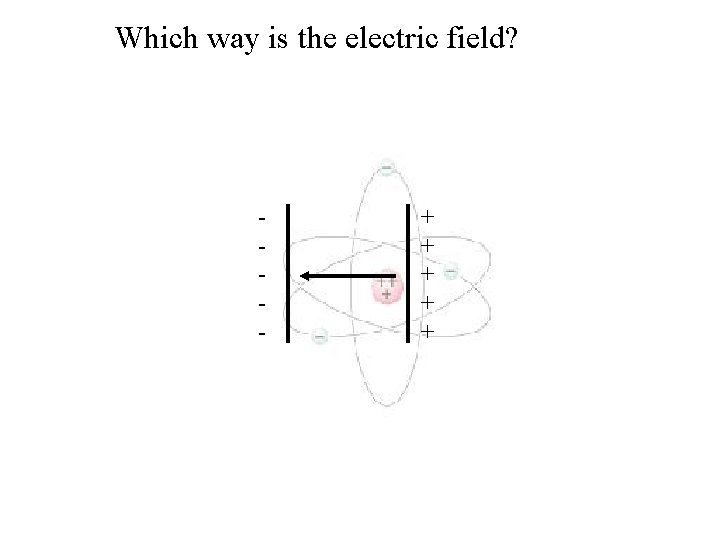Which way is the electric field? - + + +Which way is the electric field? +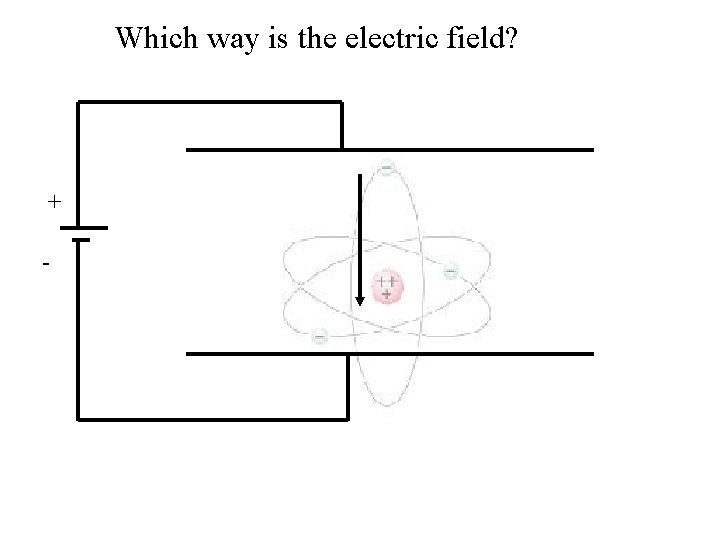Which way is the electric field? + -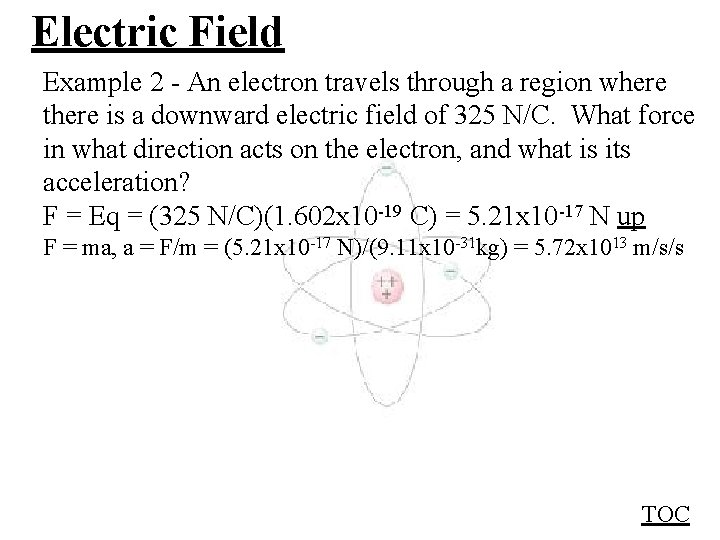Electric Field Example 2 - An electron travels through a region where there is a downward electric field of 325 N/C. What force in what direction acts on the electron, and what is its acceleration? F = Eq = (325 N/C)(1. 602 x 10 -19 C) = 5. 21 x 10 -17 N up F = ma, a = F/m = (5. 21 x 10 -17 N)/(9. 11 x 10 -31 kg) = 5. 72 x 1013 m/s/s TOC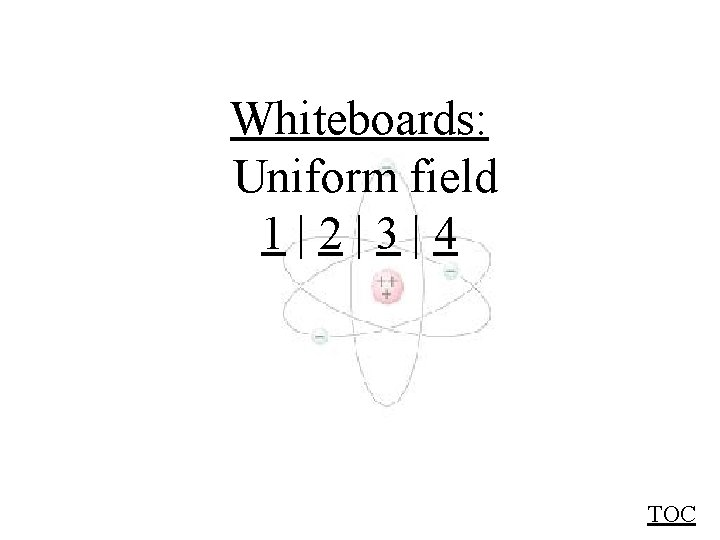Whiteboards: Uniform field 1|2|3|4 TOC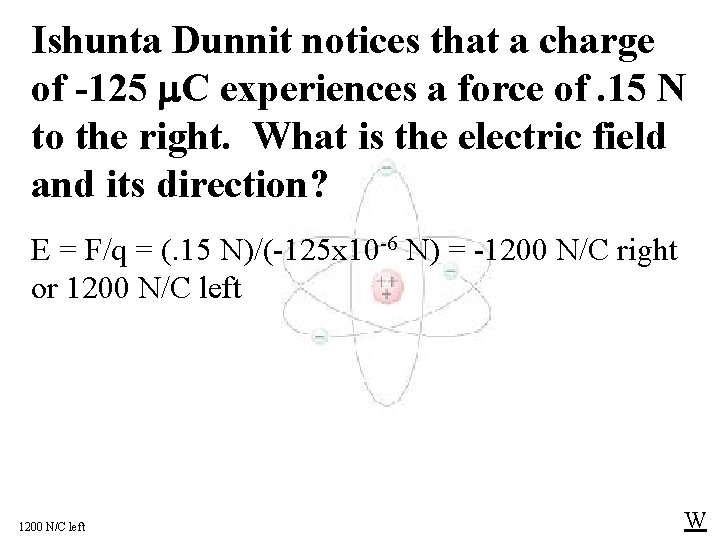Ishunta Dunnit notices that a charge of -125 C experiences a force of. 15 N to the right. What is the electric field and its direction? E = F/q = (. 15 N)/(-125 x 10 -6 N) = -1200 N/C right or 1200 N/C left WDoan Botherme places a +12 m. C charge into an upward 160 N/C electric field. What force in what direction does it experience? E = F/q, q = 12 x 10 -3 C, E = 160 N/C up F = 1. 92 = 1. 9 N up WAlfred O. Dadark is on a planet where a mass of 0. 12 kg experiences a downward force of 7. 80 N. What is the gravitational field on the surface of this planet? g = F/m, m = 1. 12 kg, E = 7. 80 N down g = (7. 80 N down)/(0. 12 kg) = 65 N/kg down WTelly Vishun places an unknown charge into a known upward electric field of 612 N/C, and the charge experiences a downward force of. 851 N. What is the charge? E = F/q, E = 612 N/C up, F =. 851 N down q = -. 0013905 C = -1. 39 m. C WSal F. Hone levitates a. 00125 kg ball with an upward electric field of 590 N/C. What is the charge on the ball? (Hint gravity = electrical force) Eq = mg E = F/q, F = Eq, F = mg, m =. 00125 kg, g = 9. 80 N/kg, E = 590 N/C q = 2. 07627 E-05 = +20. 8 C WElectric fields around point charges Direction of E field is: Away from positive Toward negative TOC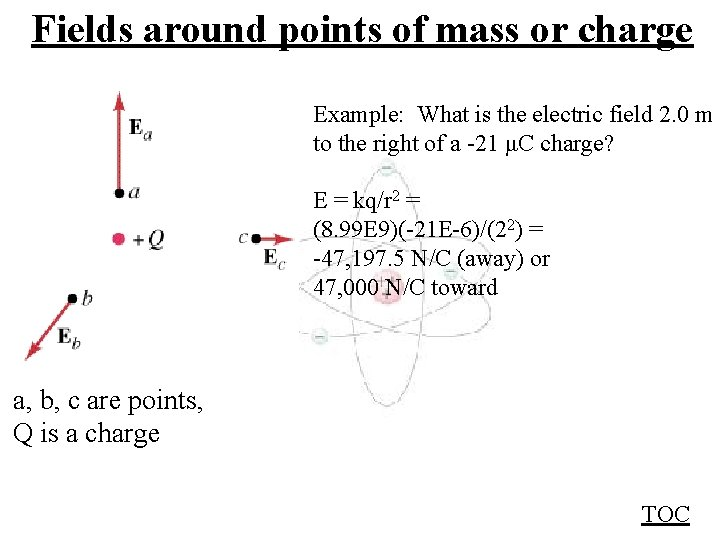Fields around points of mass or charge Example: What is the electric field 2. 0 m to the right of a -21 μC charge? E = kq/r 2 = (8. 99 E 9)(-21 E-6)/(22) = -47, 197. 5 N/C (away) or 47, 000 N/C toward a, b, c are points, Q is a charge TOCWhiteboards: Field relative to points 1|2|3 TOC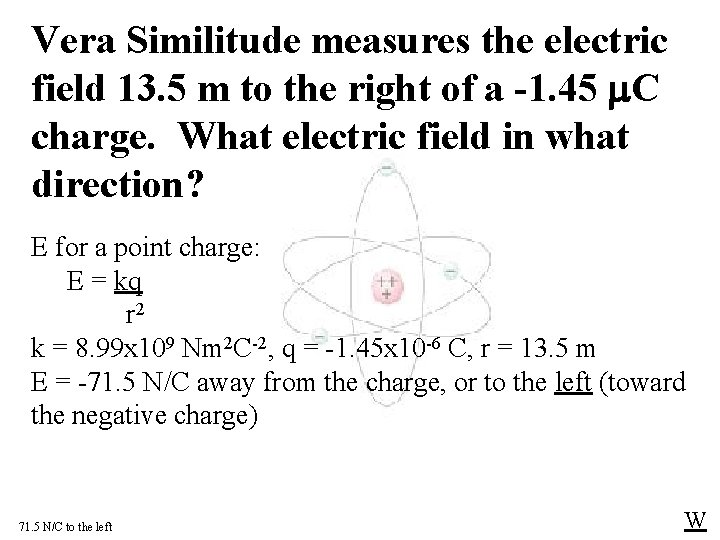Vera Similitude measures the electric field 13. 5 m to the right of a -1. 45 C charge. What electric field in what direction? E for a point charge: E = kq r 2 k = 8. 99 x 109 Nm 2 C-2, q = -1. 45 x 10 -6 C, r = 13. 5 m E = -71. 5 N/C away from the charge, or to the left (toward the negative charge) 71. 5 N/C to the left WVesta Buhl measures an electric field of 2, 120 N/C, 67 cm from a charge of unknown value. The electric field is away from the charge. What is the charge? E for a point charge: E = kq r 2 k = 8. 99 x 109 Nm 2 C-2, E = 2, 120 N/C, r =. 67 m q = 1. 06 x 10 -7 C = +. 11 C. It is a positive charge as the E-field is away from it +. 11 C WAmelia Rate measures a gravitational field of 3. 4 N/kg. What distance is she from the center of the earth? (Me = 5. 98 x 1024 kg. ) g for a point mass: g = Gm r 2 G = 6. 67 x 10 -11 Nm 2 kg-2, g = 3. 4 N/kg, m = 5. 98 x 1024 kg r = 10831137. 03 m = 10. 8 x 106 m (re = 6. 38 x 106 m) 1. 1 x 107 m WTara Bull measures an electric field of 10. N/C what distance from an electron? E for a point charge: E = kq r 2 k = 8. 99 x 109 Nm 2 C-2, E = 10 N/C, q = 1. 602 x 10 -19 C r = 1. 20008 x 10 -5 m = 12 m W+12. 5 C x A 72. 1 cm -187 C C 11. 2 cm What is the electric field at the x? (Magnitude and direction) EA = kq. A = 8. 958 E 6 N/C to the left (away from +) r 2 EC = kq. C = 2. 423 E 6 N/C to the right (toward -) r 2 = - 8. 958 E 6 N/C + 2. 423 E 6 N/C = -6. 54 E 6 N/C (Left) 6. 54 E 6 N/C to the left WEach grid is a meter. If charge A is -14. 7 μC, and charge B is +17. 2 μC, calculate the electric field at the origin: y A x Bmag angle x y A 7773. 7 165. 96 -7541. 6 1885. 4 B 19328. 5 45 13667. 3 6125. 7 15552. 7 Mag 16, 715 N/C angle 68. 5º (trig angle)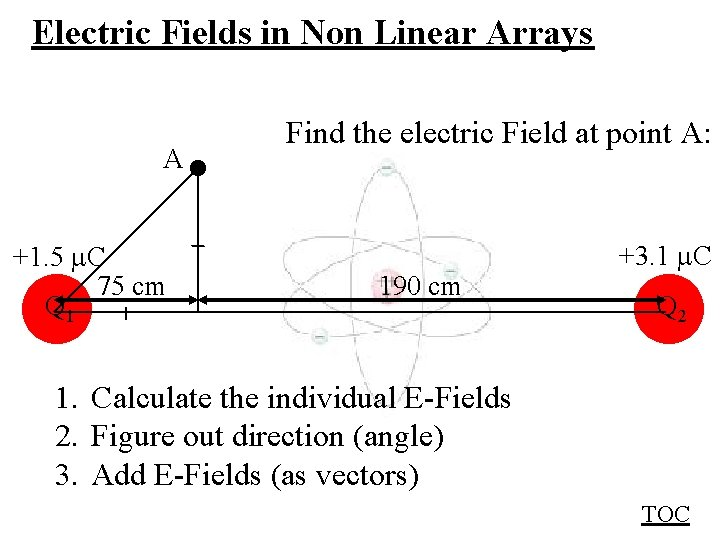Electric Fields in Non Linear Arrays A +1. 5 C 75 cm Q 1 Find the electric Field at point A: 190 cm +3. 1 C Q 2 1. Calculate the individual E-Fields 2. Figure out direction (angle) 3. Add E-Fields (as vectors) TOC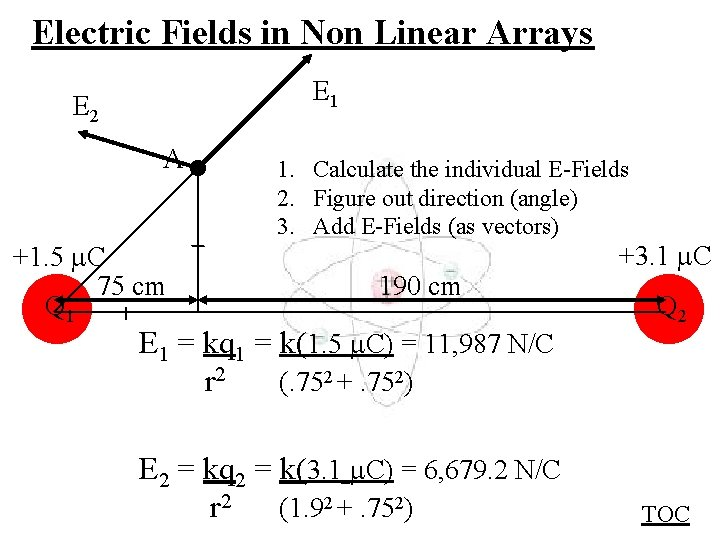Electric Fields in Non Linear Arrays E 1 E 2 A +1. 5 C 75 cm Q 1 1. Calculate the individual E-Fields 2. Figure out direction (angle) 3. Add E-Fields (as vectors) 190 cm E 1 = kq 1 = k(1. 5 C) = 11, 987 N/C r 2 (. 752 +. 752) E 2 = kq 2 = k(3. 1 C) = 6, 679. 2 N/C r 2 (1. 92 +. 752) +3. 1 C Q 2 TOCElectric Fields in Non Linear Arrays E 2 = 6, 679. 2 N/C A 2 +1. 5 C 75 cm Q 1 E 1 = 11, 987 N/C 1 190 cm Figuring out direction: 1 = 45 o 2 = Tan-1(. 75/1. 9) = 21. 54 o T = 180 -21. 54 = 158. 46 o Really hoping I don’t need to show any more… +3. 1 C Q 2 TOC